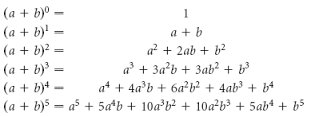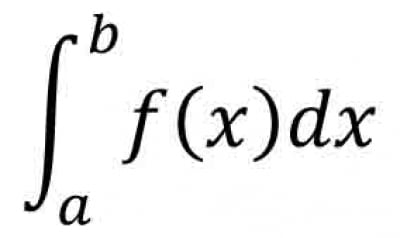# Core 2 Key Points

?
• Created by: LShan261
• Created on: 25-04-15 18:50

## Algebra and functions

• If f(x) is a polynomial and f(a) = 0, then (x-a) is a factor of f(x)
• If f(x) is a polynomial and f(b/a) = 0, then (ax-b) is a factor of f(x)
• If a polynomial f(x) is / by (ax-b) then the remainder is f(b/a)
1 of 11

## The sine and cosine rule

• Sine rule
• a/sinA = b/sinB = c/sinC
• 2 angles & length of opposite side --> unknown side
• 2 sides & opposite angle --> unknown angle
• Cosine rule
• a^2 = b^2 + c^2 - 2bccosA or b^2 = a^2 + c^2 - 2accosB or c^2 = a^2 + b^2 - 2abcosC
• 2 sides & angle between --> unknown side
• 3 lengths --> unknown angle
• Rearranged form of cosine rule
• unknown angle
• cosA = b^2 + c^2 - a^2/2bc or cosB = a^2 + c^2 - b^2/2ac or cosC = a^2 + b^2 - c^2/2ab
• area of a triangle
• 1/2absinC
• length of 2 sides (a and b) & angle C
2 of 11

## Exponential and logarithms

• function y = a^x, or f(x) = a^x --> a = constant --> exponential function
• logan = x --> a^x = n --> a = base
• loga1 = 0
• logaa = 1
• log10x --> logx
• Laws of logarithms
• logaxy = logax + logay --> multiplication law
• loga(x/y) = logax - logay --> division law
• loga(x)^k = klogax --> the power law
• From the power law
• loga(1/x) = -logax
• Solve equation e.g. a^x = b by 1st taking logarithms (to base 10) of each side
• Change of base rule
• logax = logbx/logba
• From the change of base rule
• logab = 1/logba
3 of 11

## Coordinate geometry in the (x,y) plane

• Mid-point of (x1,y1) and (x2,y2)
• (x1 + x2/2, y1 + y2/2)
• distance d between (x1, y1) and (x2, y2)
• d=√[(x2-x1)^2 + (y2-y1)^2]
• Equation of the circle centre
• (x-a)^2 + (y - b)^2 = r^2
• Chord = line that joins two points on circumference of a circle
• The perpindicular from the centre of  circle to chord --> bisects cord
• Angle in a semicircle --> right angle
• Tangent = line that meets circle @ 1 point only
• Angle btwn tangent & radius = 90 degrees
4 of 11

## The binomial expansion

• use Pascal's Triangle to multiply out bracket
• The binomial expansions is
•5 of 11

## Radian measure & its application

• If arc AB has length, r, then angle AOB is 1 radian (1c or 1rad)
• Radian = angle subtended @ centre of circle by an arc whose length is = to that of the radius of the circle
• 1 radian = 180 degrees/π
• Length of an arc
• l = rØ   (Ø = theta)
• Area of a sector
• 1/2r^2Ø
• Area of a segment
• 1/2r^2(Ø - sinØ)
6 of 11

## Geometric sequences and series

• next term --> multiply by common ratio
• nth term
• ar^n-1 --> a=1st term   r=common ratio
• Sum to n terms
• Sn = a(1-r^n)/1-r or Sn = a(r^n - 1)/r-1
• Sum to infinity
• S∞ = a/1-r
7 of 11

## Graphs of trigonometric functions

• the x-y plane / into quadrants
• for all values of Ø, definitions of sin Ø, cos Ø and tan Ø are taken to be
• sinØ = y/r
• cos Ø = x/r
• tan Ø = y/x
• x and y = coordinates     r = radius
• 1 st quadrant --> Ø = acute
• All functions = +ve
• 2nd quadrant --> Ø = obtuse
• Sine = +ve
• 3rd quadrant --> Ø = reflex
• 180 degrees < Ø < 270 degrees
• Tangent = +ve
• 4th quadrant --> Ø = reflex
• 270 degrees < Ø < 360 degrees
• Cosine = +ve
• Sine & cosine have a period of 360 degrees (2π rad) --> tangent period of 180 degrees (π rad)
8 of 11

## Differentiation

• Increasing function f(x) in interval (a.b). f'(x) > 0 in the interval a≤x≤b
• Decreasing function f(x) in the interval (a,b), f'(x) < 0 in the interval a≤x≤b
• Maximum point = where f(x) stops increasing & begins to decrease
• Minimum point = f(x) stops decreasing & begins to increase
• Point of inflexion = where the m is @ a max/min value in the neighbourhood of points
• Stationary point = 0 m --> max/min/inflexion
• Find the coordinates of a stationary point
• find dy/dx --> solve f'(x) = 0 --> sub into y = f(x) to find corresponding values of y
• Determining the nature of a stationary point
• dy/dx = 0 & d^2y/dx^2 > 0 --> minimum point
• dy/dx = 0 & d^2y/dx^2 < 0 --> maximum point
• dy/dx = 0 & d^2y/dx^2 = 0 --> max/min/inflexion
• dy/dx = 0 & d^2y/dx^2 = 0 BUT d^3y/dx^3 ≠ 0 --> point of inflexion
• need to find the max/min point
• establish formula for y in terms of x
• differentiate
• derived function = 0
• find x then y
9 of 11

## Trigonometrical identities & simple equations

• tan Ø = sin Ø/cos Ø
• sin^2Ø + cos^2Ø = 1
• sinx = k
• 1st solution --> a = sin^-1(k)     (a = alpha)
• 2nd solution --> (180 degrees - a) or (π - a), if working in radians
• cosx = k
• 1st solution --> a = cos^-1(k)
• 2nd solution --> (360 degrees - a) or (2π - a)
• tanx = k
• 1st solution --> a = tan^-1(k)
• 2nd solution --> (180 degrees + a) or (π + a)
10 of 11

## Integration

•= f(b) - f(a)

• Area beneath curve w/ equation y = f(x) & between line x=a and x=b
• Area between a line (equation y1) and a curve (equation y2) is given by
• Same as above BUT f(x) = (y1 - y2)
• Trapezium rule
•• h = b-a/n and yi = f(a + ih)
11 of 11